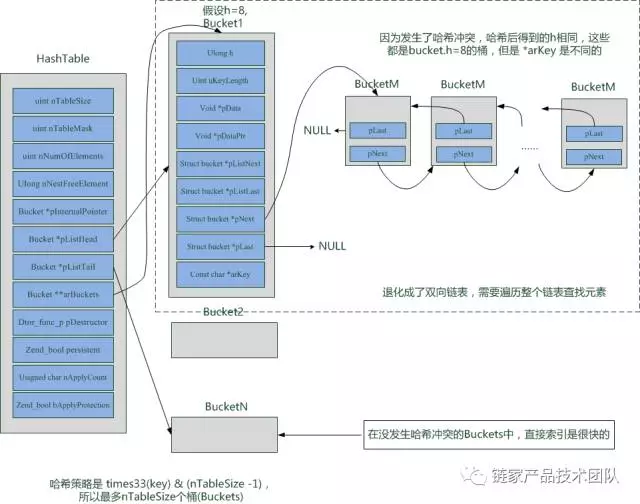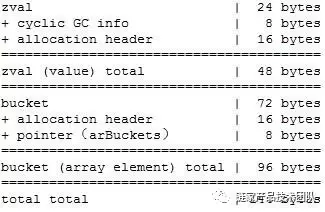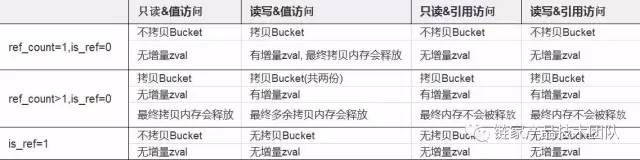# 从 foreach 方法引出的 PHP 内存分析PHP 代码中 Foreach 结构随处可见，我们在使用时，是否了解其行为呢？我们这篇文章通过一些例子来分析下 Foreach 结构的内存行为。如果你想了解 PHP 内存相关的内容，不妨把这篇文章作为一个参考。

## 问题

$arr = [‘a’,‘b’,‘c’,‘d’]; 非引用方式: foreach($arras$key =>$value) {

$arr[$key] = $value .$value;

}

foreach($arras &$item) {

$item =$item . $item; } 对此，老司机们建议我们采用非引用方式，主要原因是变量的作用域。下面我们来看一个具体案例。 ## 变量作用域 老司机们建议我们：在使用引用方式去遍历数组时，最好遍历结束后显式地 unset 掉该引用。原因是变量的作用域是整个函数，如果不 unset 掉该引用，在这个函数内其他地方操作这个引用时会引起冲突。下面我们来看这段代码：$arr = [‘a’,‘b’,‘c’,‘d’];

foreach($arras &$item) {

$item =$item . $item; } foreach($arras$item) { var_dump($item);

}

var_dump($item); 结果为： string(2)“aa” string(2)“bb” string(2)“cc” string(2)“dd” string(2)“aa” string(2)“bb” string(2)“cc” string(2)“cc” string(2)“cc” 第一次的遍历打印了 aa,bb,cc,dd 比较容易理解，但是第一次遍历完成后 $item 保留下来了，而且指向$arr 的最后一个元素。这样在第二次循环中，实际的行为是将 $arr 的每个元素依次赋值给了$item。具体的行为是： 第一次：’aa’ => $item$arr = [‘aa’, ‘bb’, ‘cc’, ‘aa’] 第二次：’bb’ => $item$arr = [‘aa’, ‘bb’, ‘cc’, ‘bb’] 第三次：’cc’ => $item$arr = [‘aa’, ‘bb’, ‘cc’, ‘cc’] 第四次：’cc’ => $item$arr = ‘aa’, ‘bb’, ‘cc’, ‘cc’] 最后$item 指向的值是’cc’，用 xdebug_debug_zval 方法可以看到每个元素的引用情况，大家可以自行验证。

## 内存消耗

### Array 内存结构

$mem_start = memory_get_usage();$arr = range(0,(1<<20) - 1);

$mem_end = memory_get_usage(); var_dump(($mem_end -$mem_start)/1024/1024); 结果是: float(144.00043487549) 注：这里计算的是 Array 实际占用内存，不包含已分配但是没有被占用的内存，详情参考 memory_get_usage 的文档。 为什么是 144MB 而不是 8MB 呢？我们要从 Array 的结构入手开始分析。 ### 哈希表 PHP 的 Array 是基于哈希表实现的，那么哈希表长什么样呢？先看下面这张图（参考 zend_hash.h）关于哈希表的定义，请参 zend_hash.h(55-84 行)，对于哈希表，我们需要记住以下几点： • nTableSize 指的是哈希表的长度，范围是 8 到 1 << 31，当如果进行一次操作后发现元素个数大于 nTableSize，长度会变为 nTableSize * 2。• nNumOfElements 指的是哈希表里面实际存储了多少元素，count 方法使用的就是这个字段 (zend_hash.c:1053-1058 行)。 • pInternalPointer 是用来做内部遍历用，指向当前的元素，reset()，current()，prev()，next()，foreach()， end() 等方法会修改这个指针。 • pListHead 和 pListTail 指向的是内部元素的头指针和尾指针，只有当 HashTable 的结构发生变化时这两个指针才会发生变化。 • arBuckets 指向存储元素 (Bucket) 的数组，里面存储的是指向 Bucket 的指针。 在 PHP 中，每个 Array 其实就是一个哈希表! ### Bucket typedefstruct bucket { ulong h; // 实际的哈希值，如果 key 是 int 类型的，那么 hash 就是 key uint nKeyLength; //key 的长度 (string 类型的 key 时才有用) void *pData; // 指向 data 的指针 void *pDataPtr; // 当 data 是指针类型时，为了避免内存碎片，直接将 data 存放到这里 struct bucket *pListNext; // 剩下几个是 bucket 指针 struct bucket *pListLast; struct bucket *pNext; struct bucket *pLast; constchar *arKey; //key 值 (string 类型的才有用) } Bucket; 关于 Bucket，我们需要理解以下内容： • Bucket 存储的是结构而不是实际的值，相当于在哈希表和实际值之间的映射关系。通过哈希运算，我们先找到对应的 Bucket，然后再从 Bucket 里面找到指向实际值的指针 (pData)，最后一步取出实际的值。 • Bucket 的大小是 ulong(8)+uint(4)+ 指针 (8*7) =68byte，加上对齐，所以实际是 72byte。 • 通过对 Bucket 结构的分析我们知道：每个 Bucket 只能存储一个 Array 元素。 ### zval PHP 中存储值的最基本元素就是 zval。在看 zval 之前，我们先看 zvalue。zvalue 定义如下 (参考 zend.h 321-330 行)： typedefunion _zvalue_value { long lval; /*long value */ double dval; /*double value */ struct { char *val; int len; } str; HashTable *ht; /*hash table value */ zend_object_value obj; } zvalue_value; • 这是 C 语言里面的 union 类型，外部可以通过不同属性获取不同类型。zvalue->lval 拿到的是 long 类型，zvalue->ht 拿到的是指向哈希表的指针。 • 这个 zvalue 结构占用的空间是 max(long, double, struct, pointer, zend_object_value)=max(8, 8, 12,8, 12)，加上对齐，实际占用 16byte。(注：zend_object_value 长度是 12byte) 我们再看 zval 的定义 (zend.h 332-338 行)。 struct _zval_struct { /* Variable information */ zvalue_value value; /* value */ zend_uint refcount__gc; zend_uchar type; /* active type */ zend_uchar is_ref__gc; }; 我们看到除了 zvalue，zval 中还包含了 GC(Garbage Collection) 的内容：比如说被引用次数 refcount_gc，是否被引用 is_ref_gc，所以总的大小是：16+4+1+1=22byte，对齐之后是 24byte。 PHP5.3 之后，对于循环引用引入了新的垃圾回收机制。这里先不介绍 GC 的细节 (参考 GC)，只是要说明引入 GC 增加了实际存储的空间。（参考 zend_gc.h 91-97 行） typedefstruct _zval_gc_info { zval z; union { gc_root_buffer *buffered; struct _zval_gc_info *next } u; } zval_gc_info; 还是老套路，union 的实际大小是 max(pointer, pointer) = max(8, 8) = 8 byte，所以包装好的 zval_gc_info 实际是 32byte。 这还不够。 C/C++ 是自己管理内存的。为了让用户不直接管理内存，PHP 在内核中加入了 MM(Memory Management) 模块。具体来讲就是为每个经 MM 分配的内容增加了一个 zend_mm_block。关于内存分配，这里先略过。我们先来看 zend_mm_block 的结构 (参考 zend_alloc.c 336-342, 366-377 行)。 复制代码 typedefstruct _zend_mm_block_info {#ifZEND_MM_COOKIES size_t _cookie;#endif size_t _size; size_t _prev;} zend_mm_block_info; typedefstruct _zend_mm_block { zend_mm_block_info info;#ifZEND_DEBUG unsignedint magic;# ifdef ZTS THREAD_T thread_id;# endif zend_mm_debug_info debug;#elifZEND_MM_HEAP_PROTECTION zend_mm_debug_info debug;#endif} zend_mm_block; 这个结构的大小受很多编译参数的影响，最小是 zend_mm_block_info，也就是两个 size_t 的长度，共 16byte。其他的编译参数在我的测试机上面没有开启，这里也暂不讨论。 所以，综合以上的分析，我们可以画出 Array 每个元素的结构：通过上面的分析，我们可以看到在 64 位操作系统中，Array 的每个元素实际上是要占用 144 字节的，所以在文章最开始问题解决了：1M 的 Array 实际占用了 144MB。 ## Foreach 内存行为 那么，第二个问题来了，如果将这 1M 的 Array 每个元素存储两次，那么消耗的空间会是 288M 么？看代码： 复制代码 $count = 0;$arr = array();$mem_start = memory_get_usage();while($count < (1<<20)){$arr[] = $count;$arr[] = $count;$count += 1;}$mem_end = memory_get_usage();var_dump(($mem_end - $mem_start)/1024/1024); 结果是：float(240.00015258789) 奇怪的是内存并不是 288M，而是 240M。根据我们对 PHP 的理解，对于相同的 zval，PHP 进行了复用，复用的结果仅仅是对该 zval 的 ref_count 加 1。用 xdebug_debug_zval 分析，我们看到： arr: (refcount=1, is_ref=0)=array (0 => (refcount=2, is_ref=0)=0, …) 对于每个 zval，refcount=2。arr 作为一个单独的 zval，refcount=1。所以在这个例子中，Array 的结构被复制了两份，zval 没有发生复制，所以占用的内存是 96M+144M=240M。 咦，好像还漏了一个问题，当 Array 的元素增长时，我们不是说过哈希表的长度是指数增长的么？我们再看一个例子： 复制代码 $count = 0;$arr = array();$start = memory_get_usage();while($count < (1<<5)) {$arr[] = $count;$count += 1;    var_dump(memory_get_usage() - $start);} 结果如下： 1 int(280) 9 int(1464) 17 int(2680) 25 int(3768) 2 int(448) 10 int(1600) 18 int(2816) 26 int(3904) 3 int(584) 11 int(1736) 19 int(2952) 27 int(4040) 4 int(720) 12 int(1872) 20 int(3088) 28 int(4176) 5 int(856) 13 int(2008) 21 int(3224) 29 int(4312) 6 int(992) 14 int(2144) 22 int(3360) 30 int(4448) 7 int(1128) 15 int(2280) 23 int(3496) 31 int(4584) 8 int(1264) 16 int(2416) 24 int(3632) 32 int(4720) 第一行和第二行我们可以忽略，因为最开始有些初始化的内容，我们不做讨论。我们重点关注 3->7，8->9，10->15，16->17，18->32。我们看到 3->7，10->15，18->32 中间的数值是等差数列，差值是 136byte。8->9 的差别是 200 = 136 + 88，16->17 的差别是 264 = 136 + 816。我们知道，哈希表的默认长度是 8。当长度从 8 增长到 9 时，长度变为 16，从 16 增长到 17 时，长度变为 32。然而在这个过程中并没有为每个元素都申请 96 字节的 bucket，而是将哈希表的 arBuckets 增加两倍，因为 arBuckets 里面存放的是指向 bucket 的指针 (8byte)，所以每次 Array 增长时实际增加的大小是 8byte* 增长的长度。136byte=72+16+48 回过头来，foreach 过程中的内存行为是什么样子的呢？ 我们分两种情况来讨论内存使用：1，只读；2，读写。我们来看个例子： $arr = range(0,(1<<5) - 1); code1:$start = memory_get_usage(); foreach($arras$k => $v){ var_dump(memory_get_usage() - start); } code2:$start = memory_get_usage(); foreach($arras$k => &$v){ var_dump(memory_get_usage() -$start); } 结果是两段代码输出是一样的，迭代过程中消耗的内存都是常量，说明迭代过程中的内存开销仅仅是迭代类和变量的开销。 当有写的情况是什么样子呢？再看个例子： 复制代码 $arr = range(0,(1<<4) - 1);code1:$start = memory_get_usage();foreach($arras$k =>$v){    var_dump(memory_get_usage() - $start);$arr[$k] =$v * 2;}var_dump(memory_get_usage() - $start); code2:$start = memory_get_usage();foreach($arras$k => &$v){ var_dump(memory_get_usage() -$start);    $v =$v * 2;}

code2 同上面只读，内存增加仍旧是常量。
code1 内存增长如下：
1 int(384) 5 int(2296) 9 int(2488) 13 int(2680)
2 int(2152) 6 int(2344) 10 int(2536) 14 int(2728)
3 int(2200) 7 int(2392) 11 int(2584) 15 int(2776)
4 int(2248) 8 int(2440) 12 int(2632) 16 int(2824)
17 int(448)

$arr = range(0,(1<<4) - 1); code1：$arr2 = $arr;var_dump(xdebug_debug_zval('arr'));$start = memory_get_usage();foreach($arras$k => $v){ var_dump(memory_get_usage() -$start);}var_dump(xdebug_debug_zval('arr'));var_dump(memory_get_usage() - $start); arr: (refcount=2, is_ref=0)=array(0 => (refcount=1, is_ref=0)=0,1 => (refcount=1, is_ref=0)=1...)1 int(2072) 5 int(2072) 9 int(2072) 13 int(2072)2 int(2072) 6 int(2072) 10 int(2072) 14 int(2072)3 int(2072) 7 int(2072) 11 int(2072) 15 int(2072)4 int(2072) 8 int(2072) 12 int(2072) 16 int(2072)int(384) code2：$arr2 = $arr;var_dump(xdebug_debug_zval('arr'));$start = memory_get_usage();foreach($arras$k => &$v){ var_dump(memory_get_usage() -$start);}var_dump(xdebug_debug_zval('arr'));var_dump(memory_get_usage() - $start); arr: (refcount=1, is_ref=0)=array(0 => (refcount=1, is_ref=0)=0,1 => (refcount=1, is_ref=0)=1...)1 int(2120) 5 int(2312) 9 int(2504) 13 int(2696)2 int(2168) 6 int(2360) 10 int(2552) 14 int(2744)3 int(2216) 7 int(2408) 11 int(2600) 15 int(2792)4 int(2264) 8 int(2456) 12 int(2648) 16 int(2840)int(2840) 我们重点对比两段代码的结果，我们发现下面几个不同： • 对于$arr 本身，code1 循环前后 refcount 没有发生变化，code2 的 refcount 变为 1。

• 循环开始时，两段代码的内存都增加了很多，说明在循环开始时发生了复制动作。

• 循环结束后，code1 的内存增加了常量。code2 代码翻倍。

$arr = range(0,(1<<4) - 1); code1：$arr2 = $arr;var_dump(xdebug_debug_zval('arr'));$start = memory_get_usage();foreach($arras$k => $v){ var_dump(memory_get_usage() -$start);    $arr[$k] = $v * 2;}var_dump(xdebug_debug_zval('arr'));var_dump(memory_get_usage() -$start);arr: (refcount=2, is_ref=0)=array(0 => (refcount=1, is_ref=0)=0,1 => (refcount=1, is_ref=0)=1...)1 int(2072)   5 int(3952)    9 int(4144)   13 int(4336)2 int(3808)   6 int(4000)   10 int(4192)   14 int(4384)3 int(3856)   7 int(4048)   11 int(4240)   15 int(4432)4 int(3904)   8 int(4096)   12 int(4288)   16 int(4480)int(2840)arr: (refcount=1, is_ref=0)=array(0 => (refcount=1, is_ref=0)=0,1 => (refcount=1, is_ref=0)=2...) code2：$arr2 =$arr;var_dump(xdebug_debug_zval('arr'));$start = memory_get_usage();foreach($arras$k => &$v){    var_dump(memory_get_usage() - $start);$v = $v * 2;}var_dump(xdebug_debug_zval('arr'));var_dump(memory_get_usage() -$start);arr: (refcount=2, is_ref=0)=array(0 => (refcount=1, is_ref=0)=0,1 => (refcount=1, is_ref=0)=1...)1 int(2120)   5 int(2312)    9 int(2504)   13 int(2696)2 int(2168)   6 int(2360)   10 int(2552)   14 int(2744)3 int(2216)   7 int(2408)   11 int(2600)   15 int(2792)4 int(2264)   8 int(2456)   12 int(2648)   16 int(2840)arr: (refcount=1, is_ref=0)=array(0 => (refcount=1, is_ref=0)=0,1 => (refcount=1, is_ref=0)=2...)int(2840)

$arr = range(0,(1<<4) - 1);$arr2 = &$arr; code1：var_dump(xdebug_debug_zval('arr'));$start = memory_get_usage();foreach($arras$k => &$v){ var_dump(memory_get_usage() -$start);}var_dump(xdebug_debug_zval('arr'));var_dump(memory_get_usage() - $start); code2：var_dump(xdebug_debug_zval('arr'));$start = memory_get_usage();foreach($arras$k => $v){ var_dump(memory_get_usage() -$start);}var_dump(xdebug_debug_zval('arr'));var_dump(memory_get_usage() - $start); code3:var_dump(xdebug_debug_zval('arr'));$start = memory_get_usage();foreach($arras$k => &$v){ var_dump(memory_get_usage() -$start);    $v =$v * 2;}var_dump(xdebug_debug_zval('arr'));var_dump(memory_get_usage() - $start); code4:var_dump(xdebug_debug_zval('arr'));$start = memory_get_usage();foreach($arras$k => $v){ var_dump(memory_get_usage() -$start);    $arr[$k] = $v * 2;}var_dump(xdebug_debug_zval('arr'));var_dump(memory_get_usage() -$start);## 总结

https://mp.weixin.qq.com/s/vbkiiIPddvXbt9YYeOicBA